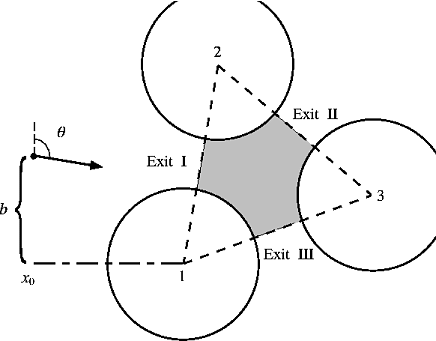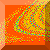The exit a particle eventually takes is sensitively dependent on its initial condition which is specified by its original location (x0 and the distance b above the x-axis) and its initial direction of approach (anglewith respect to the y-axis). The scattering region is defined as the shaded region in the diagram. We are interested in trajectories that enters the scattering region at some point and eventually leaves through one of the exits. In all subsequent computation, the following values are used: R = 1.8, L = 4.0, x0 = -4.0, and the vertices of the triangle are (0,0), (0.69,3.94), (3.76,1.37).Chaos at Maryland Home Page